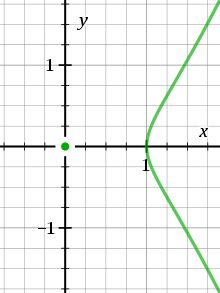# AcnodeAn acnode at the origin (curve described in text)

An acnode is an isolated point in the solution set of a polynomial equation in two real variables. Equivalent terms are "isolated point or hermit point".

For example the equationhas an acnode at the origin, because it is equivalent toandis non-negative only when≥ 1 or. Thus, over the real numbers the equation has no solutions forexcept for (0, 0).

In contrast, over the complex numbers the origin is not isolated since square roots of negative real numbers exist. In fact, the complex solution set of a polynomial equation in two complex variables can never have an isolated point.

An acnode is a critical point, or singularity, of the defining polynomial function, in the sense that both partial derivativesandvanish. Further the Hessian matrix of second derivatives will be positive definite or negative definite, since the function must have a local minimum or a local maximum at the singularity.